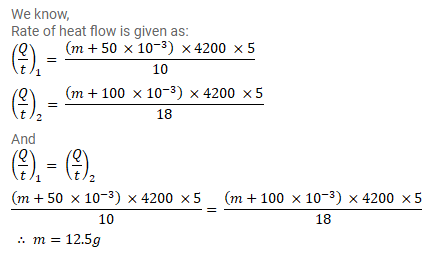# A calorimeter contains 50g of water at 50°C.Question:

A calorimeter contains $50 \mathrm{~g}$ of water at $50^{\circ} \mathrm{C}$. The temperature falls to $40^{\circ} \mathrm{C}$ in 10 minutes. When the calorimeter contains $100 \mathrm{~g}$ of water at $50^{\circ} \mathrm{C}$, it takes 18 minutes for the temperature to become $45^{\circ} \mathrm{C}$. Find the water equivalent of the calorimeter.

Solution: